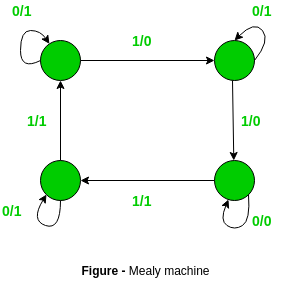GFG App
Open AppBrowser
Continue

# Difference between Mealy machine and Moore machine

Mealy Machine is defined as a machine in the theory of computation whose output values are determined by both its current state and current inputs. In this machine at most one transition is possible.
It has 6 tuples: (Q, q0, ∑, ▲, δ, λ’)

1. Q is a finite set of states
2. q0 is the initial state
3. ∑ is the input alphabet
4. ▲ is the output alphabet
5. δ is the transition function that maps Q×∑ → Q
6. ‘λ’ is the output function that maps Q×∑→ ▲

Prerequisite – Mealy and Moore Machines

The diagram is as follows:### Moore Machine:

Moore’s machine is defined as a machine in the theory of computation whose output values are determined only by its current state. It has also 6 tuples

`(Q, q0, ∑, ▲, δ, λ) `
1. Q is a finite set of states
2. q0 is the initial state
3. ∑ is the input alphabet
4. ▲ is the output alphabet
5. δ is the transition function that maps Q×∑ → Q
6. λ is the output function that maps Q → ▲

Diagram:The difference between the Mealy machine and Moore machine is as follows:

My Personal Notes arrow_drop_up